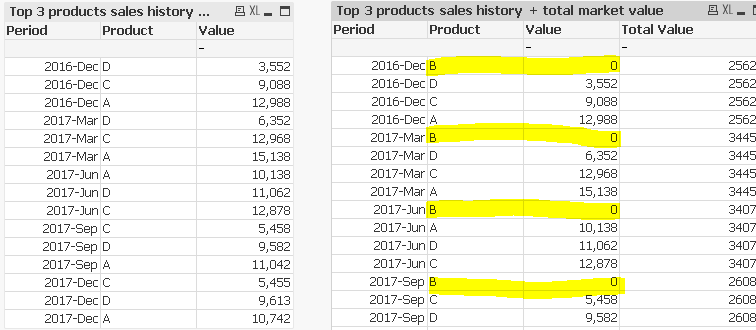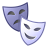# QlikView App Dev

Discussion Board for collaboration related to QlikView App Development.

Announcements
Customer & Partners, DEC. 9, 11 AM ET: Qlik Product & Strategy Roadmap Session: Data Analytics REGISTER NOW
cancel
Showing results for
Did you mean:Creator

## MAT Total + Top N

Hi everyone,

I have a table showing MAT sales of my Top 3 products on the left. I would like to add another column - total sales - to show the total sales in that period regardless of product (but only for my top 3 products). However, when I added TOTAL to my formula, it disregards my set analysis for Top 3 products and "Product B" appeared, as seen on table on the right. How do I get rid of the highlighted rows?I've attached a sample app. MAT has to be calculated on the frontend because I would like the period to be flexible + users may make other selections.  Any help would be greatly appreciated!!!

1 Solution

Accepted SolutionsChampion II

You just need to restrict your dimensions by placing the Rank condition of Top 3 outside your Pick Match

=if(AGGR(Rank(Sum(Value))<=3,_RollingMAT,Product)

,Pick(_RollingMAT,

Product={"=Rank(Sum(Value))<=3"}>}Value),

Product={"=Rank(Sum(Value))<=3"}>}Value),

Product={"=Rank(Sum(Value))<=3"}>}Value),

Product={"=Rank(Sum(Value))<=3"}>}Value),

Product={"=Rank(Sum(Value))<=3"}>}Value))

)

4 RepliesCreator II

Hi,

See if this works for you.

Thanks,

RTCreator
Author

Yes it did, thank you!

Just out of curiosity, since the IF command affects performance, do you think there's any other way to achieve the same result without sacrificing performance?Champion II

You just need to restrict your dimensions by placing the Rank condition of Top 3 outside your Pick Match

=if(AGGR(Rank(Sum(Value))<=3,_RollingMAT,Product)

,Pick(_RollingMAT,

Product={"=Rank(Sum(Value))<=3"}>}Value),

Product={"=Rank(Sum(Value))<=3"}>}Value),

Product={"=Rank(Sum(Value))<=3"}>}Value),

Product={"=Rank(Sum(Value))<=3"}>}Value),